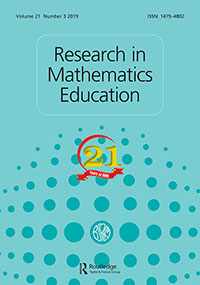# The unit of analysis in the formulation of research problems: the case of mathematical modelling at university level

 Authors Barquero, B.; Bosch, M.; Gascón, J. Abstract Taking the example of teaching mathematical modelling in university degrees, this paper focuses on how the unit of analysis adopted by different research frameworks in mathematics education transforms a teaching problem into a research one. The transformation starts by including a specific way of conceiving not only the activity of modelling but also its possible evolutions and connections with more general mathematical or scientific activities. This epistemological dimension of the research problem can be enlarged by what we call the ecological dimension, that is, the set of conditions and constraints that allow or hinder the existence of specific mathematical activities, such as modelling, as normalised school practices, in particular, at the university. The institutional perspective adopted by the Anthropological Theory of the Didactic integrates both dimensions. This integration leads to the formulation of new research questions in the domain of modelling and applications and provides new tools to address them systematically.WoS Scopus   Altmetrics Journal Research in Mathematics Education, 2019, vol. 21, no. 3, p. 314-330 Date of publication 2019-07-12 DOI https://doi.org/10.1080/14794802.2019.1624602